# RBF神经网络学习及实践

## RBF神经网络

RBF神经网络模型是1988年由Moody和Darken提出的一种神经网络结构，属于前向神经网络类型，能够以任意精度逼近任意连续函数，特别适合于解决分类问题。RBF网络是一种三层前向网络，第一层为由信号源节点组成的输入层，第二层为隐层，隐单元数视问题需要而定，隐单元的变换函数为非负非线性的函数RBF（径向基函数），第三层为输出层，输出层是对隐层神经元输出的线性组合 [ 1 ] ^{} 。网络结构大致如下图所示。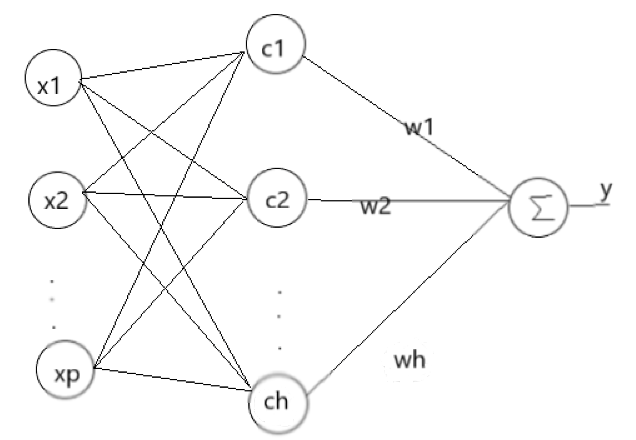R ( x p − c i ) = exp ⁡ ( − 1 2 σ 2 ∥ x p − c i ∥ 2 ) R\left(x_p-c_i\right)=\exp \left(-\frac{1}{2 \sigma^2}\left\|x_p-c_i\right\|^2\right)

y j = ∑ i = 1 h w i j exp ⁡ ( − 1 2 σ 2 ∥ x p − c i ∥ 2 ) j = 1 , 2 , ⋯   , n y_j=\sum_{i=1}^h w_{i j} \exp \left(-\frac{1}{2 \sigma^2}\left\|x_p-c_i\right\|^2\right) j=1,2, \cdots, n

σ = 1 P ∑ j m ∥ d j − y j c i ∥ 2 \sigma=\frac{1}{P} \sum_j^m\left\|d_j-y_j c_i\right\|^2

## 网络学习方法

RBF神经网络求解的参数有3个：

1. 基函数的中心；
2. 方差；
3. 隐含层到输出层的权值。

1. 自组织选取中心学习法

第一步：无监督学习过程，求解隐含层基函数的中心与方差

第二步：有监督学习过程，求解隐含层到输出层之间的权值

首先，选取h个中心做k-means聚类，对于高斯核函数的径向基，方差由公式求解：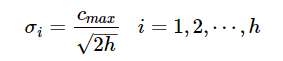其中 c m a x cmax 为所选取中心点之间的最大距离。

隐含层至输出层之间的神经元的连接权值可以用最小二乘法直接计算得到，即对损失函数求解关于 w w 的偏导数，使其等于0，可以化简得到计算公式为：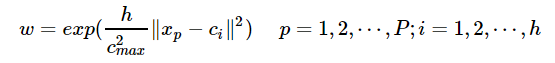2. 直接计算法

1. 有监督学习算法

## RBF神经网络特点

• 局部逼近

RBF神经网络的隐神经元采用输入向量与中心向量的距离（如欧式距离）作为函数的自变量，并使用径向基函数（如Gaussian函数）作为激活函数。神经元的输入离径向基函数中心越远，神经元的激活程度就越低（高斯函数）。RBF网络的输出与部分调参数有关，譬如，一个 w i j w_{ij} 值只影响一个 y i y_i 的输出，RBF神经网络因此具有**“局部逼近”**特性。

所谓局部逼近是指目标函数的逼近仅仅根据查询点附近的数据。而事实上，对于径向基网络，通常使用的是高斯径向基函数，函数图像是两边衰减且径向对称的，当选取的中心与查询点（即输入数据）很接近的时候才对输入有真正的映射作用，若中心与查询点很远的时候，欧式距离太大的情况下，输出的结果趋于0，所以真正起作用的点还是与查询点很近的点，所以是局部逼近。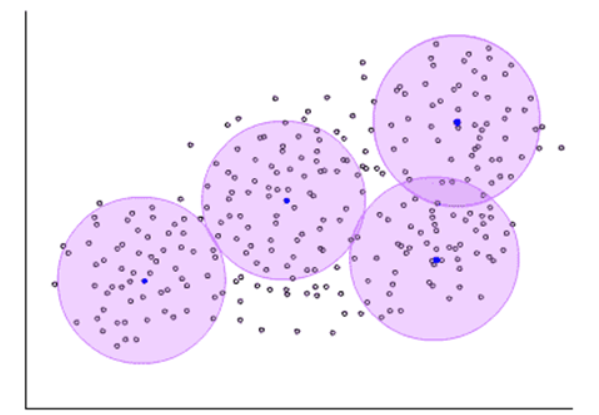• 训练速度快

使用RBF的训练速度快，一方面是因为隐含层较少，另一方面，局部逼近可以简化计算量。对于一个输入x，只有部分神经元会有响应，其他的都近似为0，对应的w就不用调参了。

## 完全内插法及其不足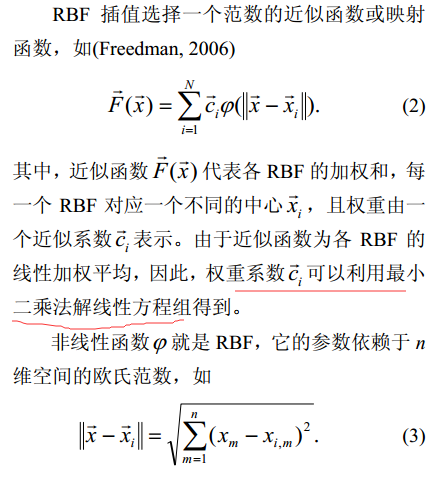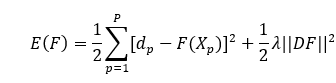## 两类径向基神经网络

### 正则化网络

• 正则化网络是一个通用逼近器，意味着，只要有足够多的隐含节点，它就可以以任意精度逼近任意多远连续函数。
• 给定一个未知的非线性函数f,总可以选择一组系数，使得网络对f 的逼近是最优的。

• 隐含节点的个数等于输入训练样本的个数，因此如果训练样本的个数Ｎ过大，网络的计算量将是惊人的，从而导致过低的效率甚至根本不可能实现。计算权值wij时，需要计算Ｎ×Ｎ矩阵的逆，其复杂度大约是O(N3)，随着Ｎ的增长，计算的复杂度迅速增大。
• 矩阵过大，病态矩阵的可能性越高。矩阵Ａ的病态是指，求解线性方程组Ａｘ = b时，Ａ中数据的微小扰动会对结果产生很大影响。病态成都往往用矩阵条件数来衡量，条件数等于矩阵最大特征值与最小特征值的比值。

## 代码实现

import time
from scipy.linalg import norm, pinv
from matplotlib import pyplot as plt
import numpy as np

class RBFN:
def __init__(self, indim, numCenters, outdim):
self.indim = indim  # 输入层维度
self.outdim = outdim  # 输出层维度
self.numCenters = numCenters  # 中心（隐层神经元）数量
self.centers = [np.random.uniform(-1, 1, indim) for i in range(numCenters)]  # 初始化中心
self.beta = 8  # 方差初始化
self.W = np.random.random((self.numCenters, self.outdim))  # 初始化权值

def _basisfunc(self, c, d):  # 激活函数定义
assert len(d) == self.indim
return np.exp(-(norm(c - d) ** 2) / (2 * self.beta ** 2))

def _calcAct(self, X):  # 计算激活函数
# calculate activations of RBFs
G = np.zeros((X.shape, self.numCenters), float)
for ci, c in enumerate(self.centers):
for xi, x in enumerate(X):
G[xi, ci] = self._basisfunc(c, x)
return G

def _calcbeta(self):  # 找到选取中心点最大值——及求解σ的值
bate_temp = np.zeros((self.numCenters, self.numCenters))  # 定义一个矩阵 隐藏层中心值确定的
for iindex, ivalue in enumerate(self.centers):
for jindex, jvalue in enumerate(self.centers):
bate_temp[iindex, jindex] = norm(ivalue - jvalue)  # 依次求解各中心层的值
return np.max(bate_temp) / np.sqrt(2 * self.numCenters)

def train(self, X, Y):
"""
:param X: 样本数*输入变量数
:param Y:样本数*输出变量数
:return:无
"""
# choose random center vectors from training set
rnd_idx = np.random.permutation(X.shape)[:self.numCenters]
self.centers = [X[i, :] for i in rnd_idx]
# print("center", self.centers)
self.beta = self._calcbeta()  # 根据样本中心计算σ值
# print(self.beta)
# calculate activations of RBFs
G = self._calcAct(X)
# print(G)
# calculate output weights (pseudoinverse)
self.W = np.dot(pinv(G), Y)  # 伪逆法直接计算得到权值

def test(self, X):
""" X: matrix of dimensions n x indim """
G = self._calcAct(X)
Y = np.dot(G, self.W)
return Y

def gen_data(x1, x2):
y_all = np.sin(np.pi * x2 / 2) + np.cos(np.pi * x2 / 3)
y_sample = np.sin(np.pi * x1 / 2) + np.cos(np.pi * x1 / 3)
# set y and add random noise
# y_sample += np.random.normal(0, 0.1, y_sample.shape)
return y_sample, y_all

if __name__ == '__main__':
num_sample = 30
# 数据生成
x1 = np.linspace(-10, 10, num_sample).reshape(-1, 1)
x2 = np.linspace(-10, 10, (num_sample - 1) * 20 + 1).reshape(-1, 1)
y_sample, y_all = gen_data(x1, x2)

# Enable interactive mode.
plt.ion()
# Create a figure and a set of subplots.
figure, ax = plt.subplots()
# plot original data
plt.plot(x2, y_all, 'r:')
plt.ylabel('y')
plt.xlabel('x')
# plot input samples
for i in range(len(x1)):
plt.plot(x1[i], y_sample[i], 'go', markerfacecolor = 'none')
# return AxesImage object for using.
pre_lines, = ax.plot([], [])
pre_lines.set_color('k')
center_points, = ax.plot([], [])
center_points.set_color('gray')
center_points.set_marker('s')
ax.set_autoscaley_on(True)
# ax.set_xlim(min_x, max_x)
ax.grid()
# plot learned model dynamically
for n_center in range(2, num_sample):
# RBF神经网络训练预测
rbf = RBFN(1, n_center, 1)
rbf.train(x1, y_sample)
y_rec = rbf.test(x2)
# update x, y data
pre_lines.set_xdata(x2)
pre_lines.set_ydata(y_rec)
plt.title('RBFN interpolation:$y=sin(\pi x/2)+cos(\pi x/3)$ with ' + str(n_center) + ' centers')
# update centers
center_points.set_xdata(rbf.centers)
center_points.set_ydata(np.zeros(rbf.numCenters))
# Need both of these in order to rescale
ax.relim()
ax.autoscale_view()
# draw and flush the figure .
figure.canvas.draw()
figure.canvas.flush_events()
time.sleep(1)
plt.figure(1)


σ = d max ⁡ 2 n \sigma=\frac{d_{\max }}{\sqrt{2 n}}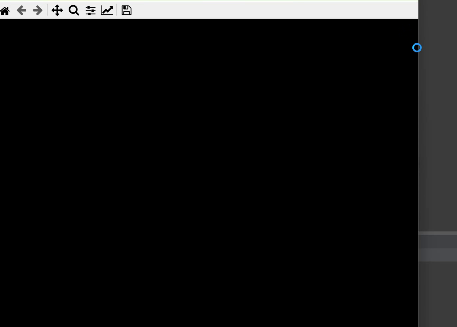## 参考

 RBF（径向基）神经网络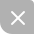UP更新不错过~
11-281752
11-061817
11-30331
10-288万+
05-211264
11-26548
05-111万+
03-233757
11-022万+
12-272782
04-261027
07-26734
10-11737
10-311332
12-109774
09-232958
10-249万+
11-259万+
05-3010万+

### “相关推荐”对你有帮助么？

•非常没帮助
•没帮助
•一般
•有帮助
•非常有帮助被折叠的  条评论 为什么被折叠?到【灌水乐园】发言MSTIFIY

¥2 ¥4 ¥6 ¥10 ¥20余额支付 (余额：-- )扫码支付获取中扫码支付点击重新获取扫码支付1.余额是钱包充值的虚拟货币，按照1:1的比例进行支付金额的抵扣。
2.余额无法直接购买下载，可以购买VIP、C币套餐、付费专栏及课程。余额充值